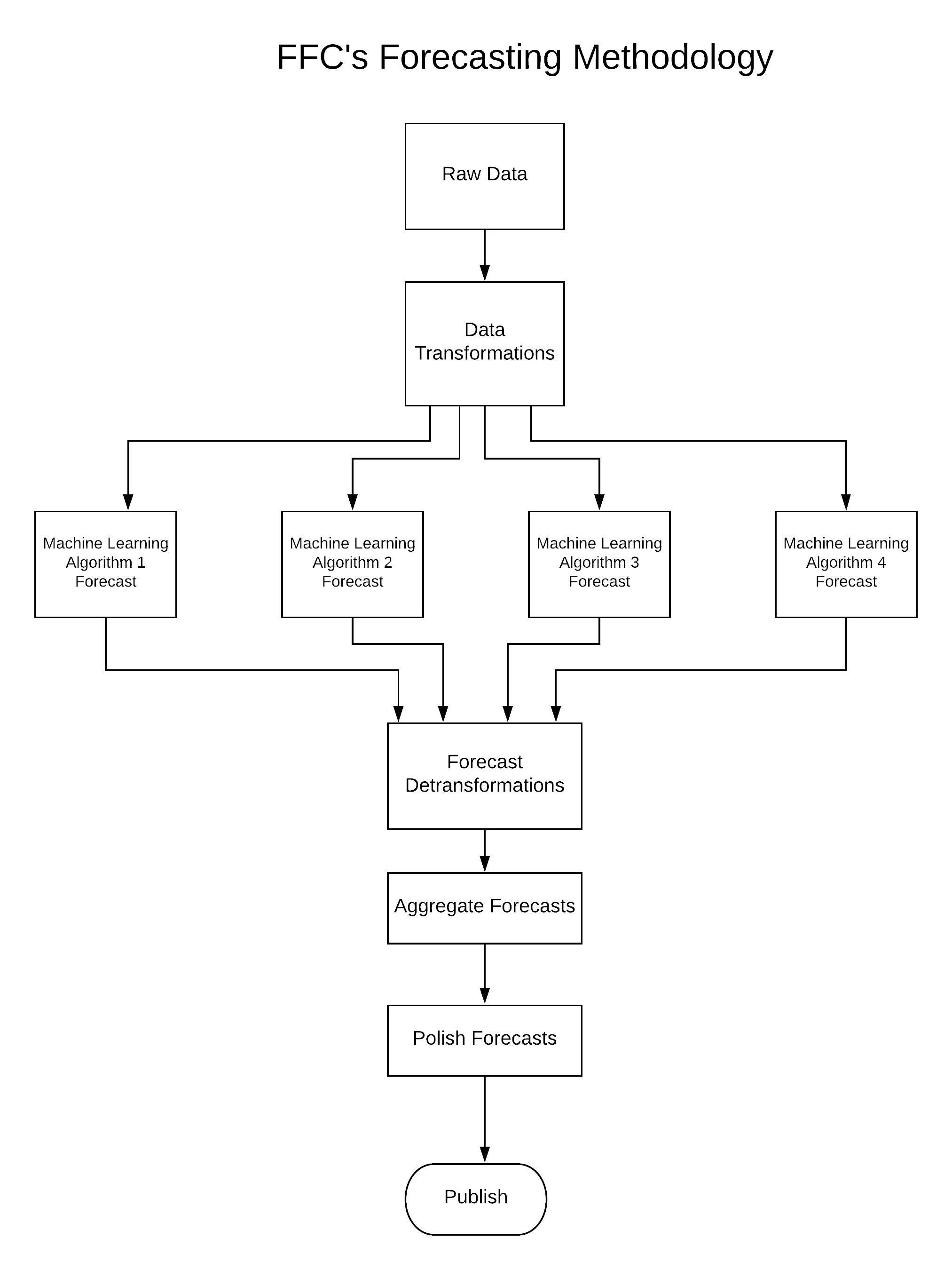The

Independent.  Objective.  Accurate.

FFC's Forecasting Methodology

## Overall Forecasting Methodology

The Financial Forecast Center employs a number of machine learning/artificial intelligence algorithms to produce all forecasts.

Below is a diagram of the overall technique of producing our forecasts with a description of each step given below the diagram.## Raw Data

FFC starts by hand picking only the finest data at the height of it's freshness. The data includes many financial and economic indicators from around the world such as stock market indexes, interest rates, currency exchange rates, commodity prices and economic indicators. FFC's comprehensive database goes back many decades.

## Data Transformations

The raw data are then transformed to improve the ability of the machine learning algorithms to forecast any specific financial indicator, or time series. For instance, FFC does not look at the raw values of the stock market indexes but the logarithm of the index values (log(x)). There are a number of different types of transformations used, and the type used depends on each individual time series. All time series (indicators) are transformed before they are presented to the AI algorithms.

## Machine Learning Algorithms

A forecast is produced by taking the transformed database above and mapping it to a specific, time-shifted target series. FFC uses numerous machine learning algorithms to map the input data to the target data.

As an example, let's take the Dow Jones Industrial Average forecast for 12 months ahead. All of the transformed time series are mapped through an AI algorithm to the DJIA time-shifted 12 months forward. This "map" is then used to take transformed data for the present month and project it to 12 months from the present. Voila, a forecast (actually, a forecast of a transform).

The number and type of algorithms used typically evolve as new, more accurate techniques are developed. It needs to be emphasized that FFC never uses a single type of algorithm to produce a forecast.

Below is a sample of our most trusted machine learning, or AI, algorithms used:

If you click on the algorithm above, it will take you to a description.

## Forecast Detransformation

Technically, the forecasts produced by each algorithm are forecasts of the transformed data. Therefore the forecasts must be transformed back to real world numbers.

## Aggregate Forecasts

A number of forecasts are produced for each month of each time series. The different forecasts are aggregated into one number for each month.

## Polish Forecasts

After the raw forecasts are produced, they are refined. This is done by analyzing the changes in a forecast time series from month to month and comparing these changes to the historical distribution. Also, forecasts of financial indicators that are very similar are compared, such as the 20 and 30 year treasury yields.

## Publish Forecasts

The forecasts are graphed and tabulated and published to www.forecasts.org.

## R, Mateys!

All forecasts are produced using the R language and computing platform.  Besides the base R environment, FFC relies heavily on quite a number of the packages available.  The whole R environment and community is an absolute necessity for the operation of the Financial Forecast Center.

## Research and Development

The Financial Forecast Center has an unhealthy obsession with the continuous improvement in the accuracy of these forecasts. About 60% of the effort at the FFC is put into research, development and testing.

Our Lab Rats work diligently at refining existing techniques and testing the performance of potential new algorithms. There is a lot of back and forth in selecting time series to include, how to transform the data and tuning/testing algorithms.